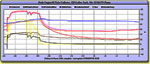Solar Hot Water Math, Formulas and ConversionsLIVE System Performance Graphs See our real-time data updated once a minute 120 - 50 = DELTA T 70F Click HERE for Solar Hot Water Math CalculationsIwalani's Voyage Around the World Weekly logs of Iwalani's three year circumnavigation written by Philip Shelton, Amy P. Wood and Stewart the Cat.The "World Voyagers" Book A true story of the three year circumnavigation by Philip Shelton, Amy Wood and Stewart the cat. From designing and building a 42 foot wooden cutter "Iwalani" to sailing around the world— this is not a watered down, sugar coated tale, but a "no holds barred" account of just what it's like to live a "dream." BTU (British Thermal Unit) Calculations 1 BTU=1 DEGREE FARENHEIT INCREASE IN ONE POUND OF WATER. 8.34 POUNDS IN ONE GALLON BTU EXAMPLE: Incoming water 50F outgoing water 120F 120 - 50 = DELTA T 70F BTU's for 1 gallon of water is 70 (delta T) * 8.34 (pounds per gallon) = 583.8 BTU's Flow Rate 2 Gallons per Minute 2 Gallons * 60 minutes = 120 Gallons per hour 120 Gallons * 583.8 BTU's per Gallon = 70,056 BTU's per Hour Solar Constant (measured outside the earth's atmosphere by satellite) is about 1.3 kilowatts (1,366 watts) per square meter (kW/m²) The maximum value is about 1 Kw (1,000 watts) per sq meter, measured at the earth's exposed surface, when the sun is directly overhead. Solar Insolation Maximum sun energy (irradiance) reaching the surface of the earth over a given time period. kWh (kiloWatt hour) to BTU examples: 1 kWh = 3,412 btu's (per square meter when the sun is directly overhead) 90,000 btu's x 0.00029307108 = 26.37 kWh Correct Flow Rates for Evacuated Tube Collector 0.026 to 0.066 Gallons per Minute per Tube 0.1 to 0.25 Liters per Minute per Tube Volume Equivalents 231 CUBIC INCHES PER GALLON 7.481 GALLONS PER CUBIC FOOT 1.02 GALLONS PER 100' OF ½" PIPE 2.29 GALLONS PER 100' OF ¾" PIPE 4.08 GALLONS PER 100' OF 1" PIPE Energy Conversions 1 kWh (kilo watt hours) = 3412.1415 BTU's 1 BTU = 0.00029307108 kWh Btu Content of Common Energy Units 1 barrel (42 gallons) of crude oil = 5,800,000 Btu 1 gallon of gasoline = 124,000 Btu (based on U.S. consumption, 2008) 1 gallon of diesel fuel = 139,000 Btu 1 gallon of heating oil = 139,000 Btu 1 barrel of residual fuel oil = 6,287,000 Btu 1 cubic foot of natural gas = 1,028 Btu (based on U.S. consumption, 2008) 1 gallon of propane = 91,000 Btu 1 short ton of coal = 19,988,000 Btu (based on U.S. consumption, 2008) 1 kilowatthour of electricity = 3,412 Btu Formulas DELTA T = TEMPERATURE DIFFERENCE BETWEEN TWO VALUES (100F-90F = 10F DELTA T) C TO F CONVERSION (C*1.8) + 32 = F F TO C CONVERSION (F - 32.0) / 1.8 = C LBS * 0.453592 = KILOGRAMS KILOGRAMS * 2.204623 = POUNDS GALLONS * 3.785412 = LITERS LITERS * 0.264272 = GALLONS QUARTS * 0.946353 = LITERS LITERS * 1.056688 = QUARTS ` ` Mouse Over the Image Above for a LARGER View of the SkyCam Picture Updated Every 15 MinutesCheck the progress of Phil's Tundra Kit PlanePaintings, Artwork and Blog of Dr. Amy Peters Wood.Building the 65' schooner "Janet May"